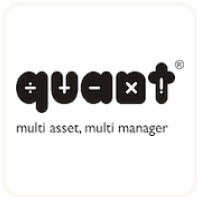Pay ₹0 for 75 days of Genius, On India's 75 years of Independence by signing up within 75 hours•Great! You have sucessfully subscribed for newsletters for investments

Subscribed email:

# Top Rated Multi Cap Funds

12  results

Funds that invest in stocks across market-caps. Let large-caps add stability, while mid-&-small caps give growth. Ideal way to invest in Equity Funds.

Show returns & current values for:
of for
Investment: 6 Lakh

## List of Top Rated Multi Cap Funds in India Ranked by Last 5 Year ReturnsConsistency
Current Value
Return (p.a)
+22.83% p.a. NA +13.23% +39.56% p.a. +18.24% NA +13.28% p.a. +6.13%
N.A. + 30.7 % p.a. N.A. + 49.43 % N.A. + 41.13 % p.a. + 116.25 % + 32.14 % p.a. + 19.69 % p.a. + 117.15 % + 23.74 % p.a.
#1 in Multi Cap ETM Rank #1 in Multi Cap
Expense 0.58%Consistency
Current Value
Return (p.a)
+16.92% p.a. NA +3.92% +29.89% p.a. +15.56% NA -0.31% p.a. +3.22%
N.A. + 22.8 % p.a. N.A. + 32.1 % N.A. + 27.87 % p.a. + 83.77 % + 18.26 % p.a. + 5.53 % p.a. + 51.11 % + 19.24 % p.a.
#2 in Multi Cap ETM Rank #2 in Multi Cap
Expense 0.32%Consistency
Current Value
Return (p.a)
+18.55% p.a. NA +4.82% +26.34% p.a. +11.59% NA +1.53% p.a. +1.17%
N.A. + 21.89 % p.a. N.A. + 23.43 % N.A. + 25.17 % p.a. + 52.78 % + 17.08 % p.a. + 4.13 % p.a. + 16.31 % + 19.27 % p.a.
#3 in Multi Cap ETM Rank #3 in Multi Cap
Expense 0.77%Consistency
Current Value
Return (p.a)
+14.84% p.a. NA +5.98% +23% p.a. +14.93% NA +1.21% p.a. +1.83%
N.A. + 18.45 % p.a. N.A. + 33.35 % N.A. + 21.82 % p.a. + 75.47 % + 16.41 % p.a. + 7.96 % p.a. + 26.69 % + 16.07 % p.a.
#5 in Multi Cap ETM Rank #5 in Multi Cap
Expense 0.51%Consistency
Current Value
Return (p.a)
+15.45% p.a. NA +4.34% +23.24% p.a. +17.7% NA -4.98% p.a. +2.42%
N.A. + 18.22 % p.a. N.A. + 34.59 % N.A. + 20.98 % p.a. + 91.67 % + 12.59 % p.a. + 2.93 % p.a. + 36.59 % + 16.16 % p.a.
#6 in Multi Cap ETM Rank #6 in Multi Cap
Expense 0.95%Consistency
Current Value
Return (p.a)
+14.16% p.a. NA +7.99% +21.83% p.a. +17.57% NA +5.88% p.a. +2.65%
N.A. + 18.12 % p.a. N.A. + 40.7 % N.A. + 24.05 % p.a. + 90.42 % + 19.98 % p.a. + 13.13 % p.a. + 40.62 % + 16.33 % p.a.
#12 in Multi Cap ETM Rank #12 in Multi Cap
Expense 0.53%Consistency
Current Value
Return (p.a)
+12.72% p.a. NA +8.33% +23.18% p.a. +17.9% NA +2.78% p.a. +4.79%
N.A. + 17.96 % p.a. N.A. + 44.43 % N.A. + 24.51 % p.a. + 100.82 % + 19.65 % p.a. + 10.9 % p.a. + 83.95 % + 17.35 % p.a.
#8 in Multi Cap ETM Rank #8 in Multi Cap
Expense 0.8%Consistency
Current Value
Return (p.a)
+13.23% p.a. NA +10.56% +19.97% p.a. +16.26% NA +6.64% p.a. +2.78%
N.A. + 17.44 % p.a. N.A. + 40.2 % N.A. + 24.4 % p.a. + 79.92 % + 21.11 % p.a. + 13.32 % p.a. + 42.95 % + 15.69 % p.a.
#18 in Multi Cap ETM Rank #18 in Multi Cap
Expense 1.08%Consistency
Current Value
Return (p.a)
+13.22% p.a. NA +7.92% +20.77% p.a. +18.24% NA -2.23% p.a. +1.96%
N.A. + 17.12 % p.a. N.A. + 39.84 % N.A. + 20.14 % p.a. + 90.6 % + 14.86 % p.a. + 7.27 % p.a. + 28.76 % + 15.95 % p.a.
#14 in Multi Cap ETM Rank #14 in Multi Cap
Expense 0.73%Consistency
Current Value
Return (p.a)
+12.16% p.a. NA +5.01% +18.73% p.a. +13.21% NA +3.93% p.a. +2.26%
N.A. + 16.42 % p.a. N.A. + 28.56 % N.A. + 21.36 % p.a. + 69.14 % + 17.01 % p.a. + 8.52 % p.a. + 33.86 % + 16.32 % p.a.
#20 in Multi Cap ETM Rank #20 in Multi Cap
Expense 0.87%Consistency
Current Value
Return (p.a)
+10.93% p.a. NA +3.78% +19.1% p.a. +14.44% NA -1.28% p.a. +1.38%
N.A. + 15.34 % p.a. N.A. + 26.85 % N.A. + 19.71 % p.a. + 70.35 % + 13.9 % p.a. + 4.84 % p.a. + 19.56 % + 15.85 % p.a.
#22 in Multi Cap ETM Rank #22 in Multi Cap
Expense 0.91%Consistency
Current Value
Return (p.a)
NA NA +3.23% +18.58% p.a. +16.26% NA -2.75% p.a. +1.75%
N.A. N.A. N.A. + 31.7 % N.A. + 17.58 % p.a. + 82.33 % + 12.84 % p.a. + 3.93 % p.a. + 25.32 % + 16.92 % p.a.
#16 in Multi Cap ETM Rank #16 in Multi Cap
Expense 0.65%
Hide comparison